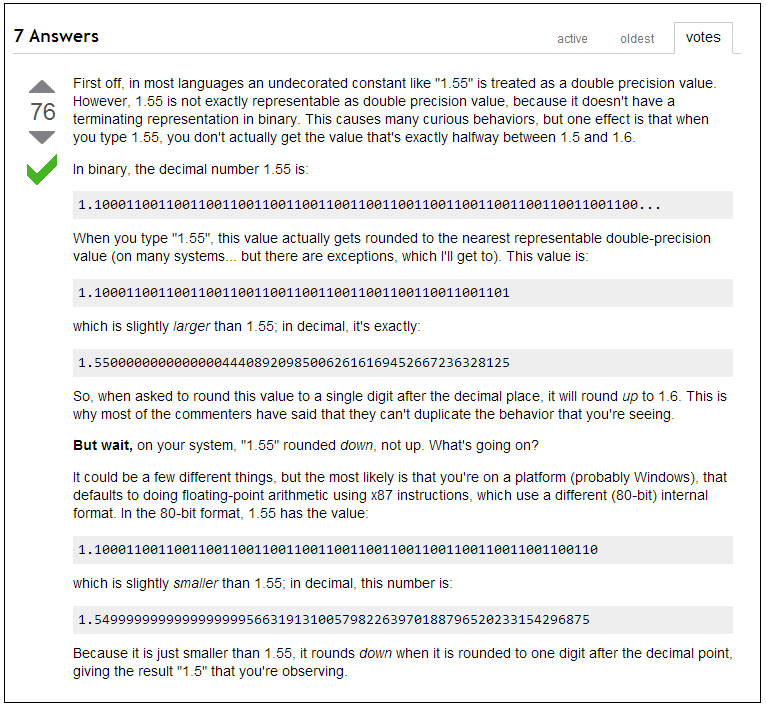Scroll

# Rounding 'error' in analysis

Follow
 Summary This article gives the explanation for a percieved rounding error that can be seen in many Analysis software packages. Applies to Askia Analyse, Askia Vista. Written for Data Processors, Analysts, Researchers, Statistical Analysts, Developers Keywords Rounding, Binary floating point, Decimal, Round up, Round down, Double precision, Programming, Computer system, Floating point arithmetic, Internal format.

The problem:

You run a table in Analyse or Vista with 3 decimal places. In one cell you see a percentage generated as 57.500%.

Now you change the decimal places on the % calculation to be 0 and re-run the table.

In the same cell you see the result as 57%. You expected it to be 58%!

The explanation:

It's because the number is converted to a representable binary floating-point number that is slightly bigger than the decimal value 57.5, so it rounds up to 58; "4.5", on the other hand, converts to a floating-point number that's slightly smaller than 4.5, so it rounds down to 4.

To really understand this, some background in computer science is probably required. Here is some recommended reading provided by the development team: http://docs.oracle.com/cd/E19957-01/806-3568/ncg_goldberg.html

This is also useful for understanding the effect:
http://stackoverflow.com/questions/2014349/why-do-programming-languages-round-down-until-6

Particularly this section below:This YouTube video is a good watch also.